##Tripole

Given a triangle ABC, draw the line L with given equation Ua*xa + Ub*xb + Uc*xc = Ud in trilinear coordinates. The trilinear coordinates xa, xb, xc denote the oriented distances from the sides of the triangle.This line passes through three points A*, B*, C* on sides BC, CA, AB respectively (see LineInTrilinears.html ). (A*, B*, C*) are correspondingly the harmonic conjugates of points (A',B',C') with respect to the pairs of points (B,C), (C,A), (A,B). Lines AA', BB', CC' intersect at a point M with trilinear coordinates (x : y : z). Below is given the calculation of the trilinears of M in terms of the coefficients Ui. M is the tripole of the line L through A*,B*,C*. L is in turn the [trilinear polar] of M with respect to the trianlge ABC.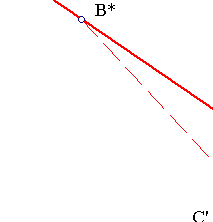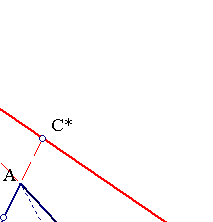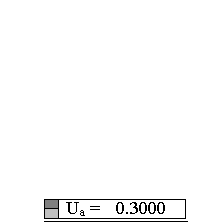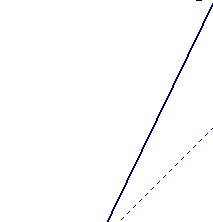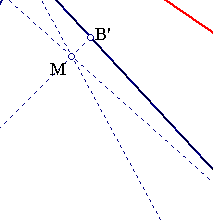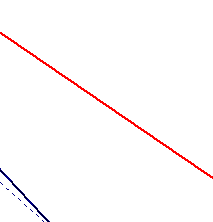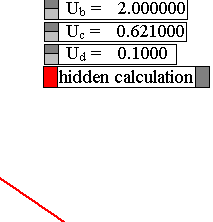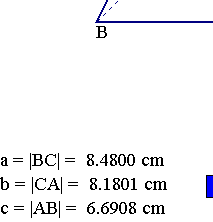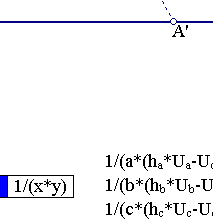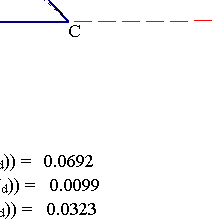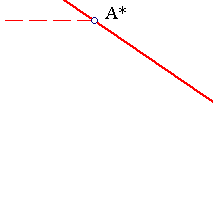The calculation of the trilinears of M (see aforementioned reference) gives:
(x : y : z ) = ( 1/(a*(ha*Ua-Ud)) : 1/(b*(hb*Ub-Ud)) : 1/(c*(hc*Uc-Ud)) ).
The relations can be solved w.r. to the Ui's expressing them as functions of the trilinears of M:
Ud = k ,
Ua = (m/(a*x) + k)/ha,
Ub = (m/(b*y) + k)/hb,
Uc = (m/(c*z) + k)/hc,
where k and the non zero m are arbitrary. In particular, for k = 0, the equation takes the form:
Ua*xa + Ub*xb + Uc*xc = 0, where (Ua : Ub : Uc) = (1/(a*x) : 1/(b*y) : 1/(c*z)).